# How to remove first (left) or last (right) characters in Excel

When working with unstructured text data in your worksheets, you often need to parse it to retrieve relevant information. This article will teach you a few simple ways to remove any number of characters from the left or right side of a text string.

## How to remove characters from left in Excel

Removing first characters from a string is one of the most common tasks in Excel, and it can be accomplished with 3 different formulas.

### Remove first character in Excel

To delete the first character from a string, you can use either the REPLACE function or a combination of RIGHT and LEN functions.

REPLACE(string, 1, 1, "")

Here, we simply take 1 character from the first position and replace it with an empty string ("").

RIGHT(string, LEN(string) - 1)

In this formula, we use the LEN function to calculate the total length of the string and subtract 1 character from it. The difference is served to RIGHT, so it extracts that many characters from the end of the string.

For example, to remove the first character from cell A2, the formulas go as follows:

`=REPLACE(A2, 1, 1, "")`

`=RIGHT(A2, LEN(A2) - 1)`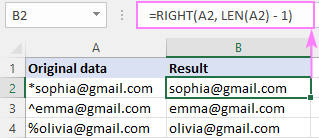### Remove characters from left

To remove leading characters from the left side of a string, you also use the REPLACE or RIGHT and LEN functions, but specify how many characters you want to delete every time:

REPLACE(string, 1, num_chars, "")

Or

RIGHT(string, LEN(string) - num_chars)

For instance, to remove first 2 characters from the string in A2, the formulas are:

`=REPLACE(A2, 1, 2, "")`

`=RIGHT(A2, LEN(A2) - 2)`

To remove first 3 characters, the formulas take this form:

`=REPLACE(A2, 1, 3, "")`

`=RIGHT(A2, LEN(A2) - 3)`

The screenshot below shows the REPLACE formula in action. With RIGHT LEN, the results would be exactly the same.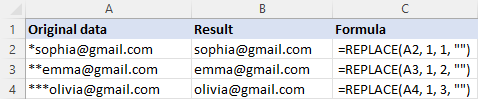### Custom function to delete first n characters

If you don't mind using VBA in your worksheets, you can create your own user-defined function to delete characters from the beginning of a string, named RemoveFirstChars. The function's code is as simple as this:

```Function RemoveFirstChars(str As String, num_chars As Long)
RemoveFirstChars = Right(str, Len(str) - num_chars)
End Function
```

Once the code is inserted in your workbook (the detailed instructions are here), you can remove first n characters from a given cell by using this compact and intuitive formula:

RemoveFirstChars(string, num_chars)

For example, to delete the first character from a string in A2, the formula in B2 is:

`=RemoveFirstChars(A2, 1)`

To strip first two characters from A3, the formula in B3 is:

`=RemoveFirstChars(A4, 2)`

To delete first three characters from A4, the formula in B4 is:

`=RemoveFirstChars(A4, 3)`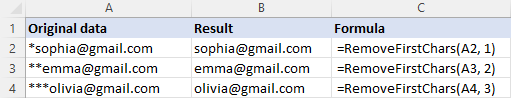To remove characters from the right side of a string, you can also use native functions or create your own one.

### Remove last character in Excel

To delete the last character in a cell, the generic formula is:

LEFT(string, LEN(string) - 1)

In this formula, you subtract 1 from the total string length and pass the difference to the LEFT function for it to extract that many characters from the beginning of the string.

For instance, to strip the last character from cell A2, the formula in B2 is:

`=LEFT(A2, LEN(A2) - 1)`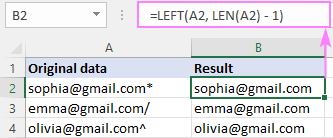### Remove characters from right

To strip off a given number of characters from the end of a cell, the generic formula is:

LEFT(string, LEN(string) - num_chars)

The logic is the same as in the above formula, and below are a couple of examples.

To remove the last 3 characters, use 3 for num_chars:

`=LEFT(A2, LEN(A2) - 3)`

To delete the last 5 characters, supply 5 for num_chars:

`=LEFT(A2, LEN(A2) - 5)`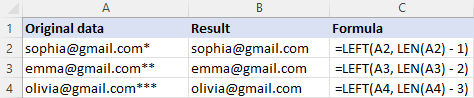### Custom function to remove last n characters in Excel

If you'd like to have your own function for removing any number of characters from right, add this VBA code to your workbook:

```Function RemoveLastChars(str As String, num_chars As Long)
RemoveLastChars = Left(str, Len(str) - num_chars)
End Function
```

The function is named RemoveLastChars and its syntax hardly needs any explanation:

RemoveLastChars(string, num_chars)

To give it a field test, let's get rid of the last character in A2:

`=RemoveLastChars(A2, 1)`

Additionally, we'll remove the last 2 characters from the right side of the string in A3:

`=RemoveLastChars(A3, 2)`

To delete the last 3 characters from cell A4, the formula is:

`=RemoveLastChars(A4, 3)`

As you can see in the below screenshot, our custom function works brilliantly!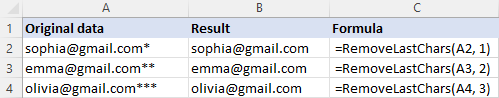## How to remove characters from right and left at once

In situation when you need to wipe out characters on both sides of a string, you can either run both of the above formulas sequentially or optimize the job with the help of the MID function.

MID(string, left_chars + 1, LEN(string) - (left_chars + right_chars)

Where:

• chars_left - the number of characters to delete from left.
• chars_right - the number of characters to delete from right.

Suppose you want to extract the username from a string like mailto:Sophia@gmail.com. For this, part of text needs to be removed from the beginning (mailto: - 7 characters) and from the end (@gmail.com - 11 characters).

Serve the above numbers to the formula:

`=MID(A2, 7+1, LEN(A2) - (7+10))`

…and the result won't keep you waiting: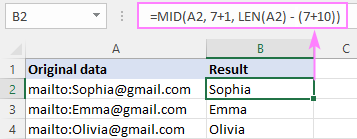To understand what's actually going on here, let's recall the syntax of the MID function, which is used to pull a substring of a certain size from the middle of the original string:

MID(text, start_num, num_chars)

The text argument does not raise any questions - it's the source string (A2 in our case).

To get the position of the first character to extract (start_num), you add 1 to the number of chars to be stripped off from left (7+1).

To determine how many characters to return (num_chars), you calculate the total of removed characters (7 + 11) and subtract the sum from the length of the entire string: LEN(A2) - (7+10)).

## Get the result as number

Whichever of the above formulas you use, the output is always text, even when the returned value contains only numbers. To return the result as a number, either wrap the core formula in the VALUE function or perform some math operation that does not affect the result, e.g. multiply by 1 or add 0. This technique is especially useful when you want to calculate the results further.

Suppose you've removed the first character from cells A2:A6 and want to sum the resulting values. Astonishingly, a trivial SUM formula returns zero. Why's that? Obviously, because you are adding up strings, not numbers. Perform one of the below operations, and the issue is fixed!

`=VALUE(REPLACE(A2, 1, 1, ""))`

`=RIGHT(A2, LEN(A2) - 1) * 1`

`=RemoveFirstChars(A2, 1) + 0`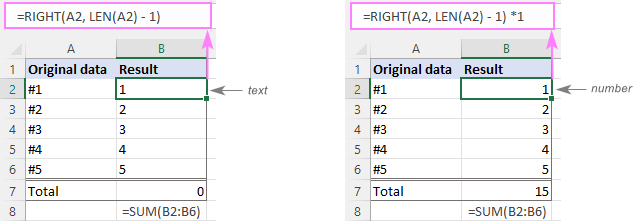## Remove first or last character with Flash Fill

In Excel 2013 and later versions, there is one more easy way to delete the first and last characters in Excel - the Flash Fill feature.

1. In a cell adjacent to the first cell with the original data, type the desired result omitting the first or last character from the original string, and press Enter.
2. Start typing the expected value in the next cell. If Excel senses the pattern in the data you are entering, it will follow the same pattern in the rest of the cells and display a preview of your data without the first / last character.
3. Just hit the Enter key to accept the preview.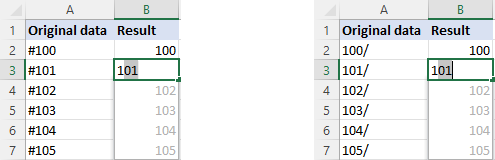## Remove characters by position with Ultimate Suite

Traditionally, the users of our Ultimate Suite can handle the task with a few clicks without having to remember a handful of various formulas.

To delete the first or last n characters from a string, this is what you need to do:

1. On the Ablebits Data tab, in the Text group, click Remove > Remove by Position.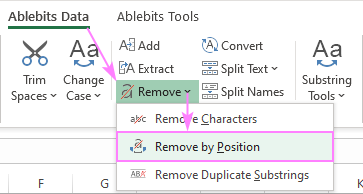2. On the add-in's pane, select the target range, specify how many characters to delete, and hit Remove.

For example, to remove the first character, we configure the following option: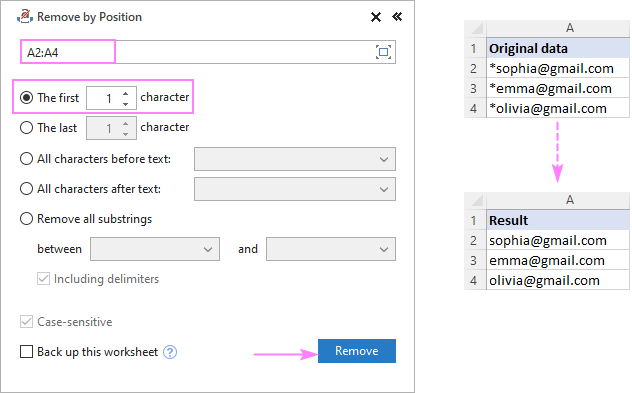That's how to remove a substring from left or right in Excel. I thank you for reading and look forward to seeing you on our blog next week!

Remove first or last characters - examples (.xlsm file)
Ultimate Suite - trial version (.zip file)

## You may also be interested in

### 3 comments to "How to remove first (left) or last (right) characters in Excel"

1.shaji shaikh says:

Thank you for the tutorial, it really helped me a lot.

2.Hrvoje Bosnar says:

Formula work with ; and not with , in Office 365

•Svetlana Cheusheva says:

Hi Hrvoje,

That depends on the List separator set in your Windows Regional settings. In the USA, UK and some other English-speaking countries, it's a comma. In most European countries, the default list separator is a semicolon because a comma is used as the decimal point.